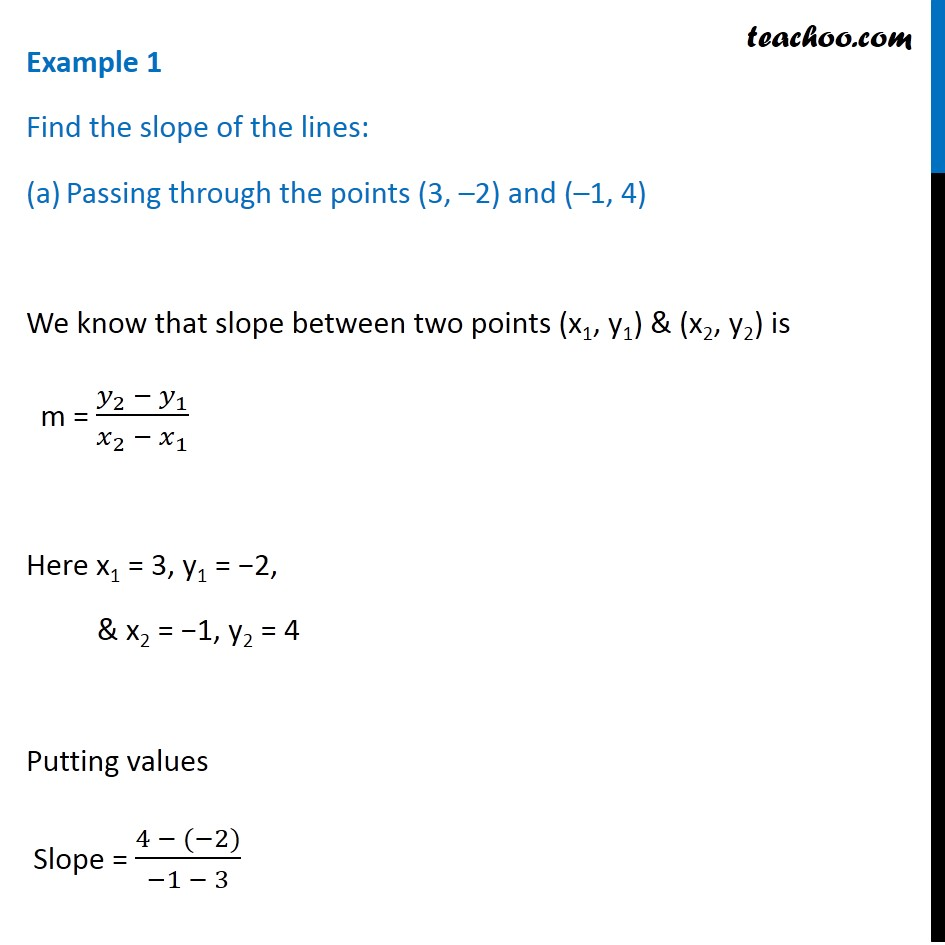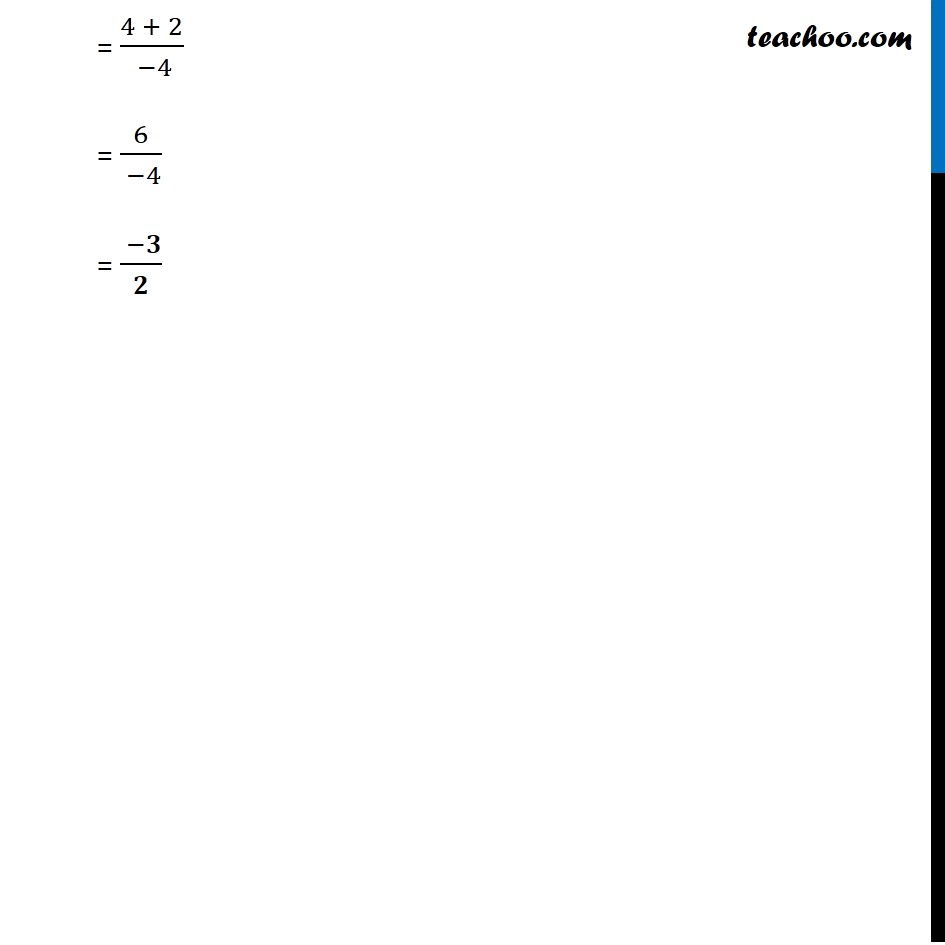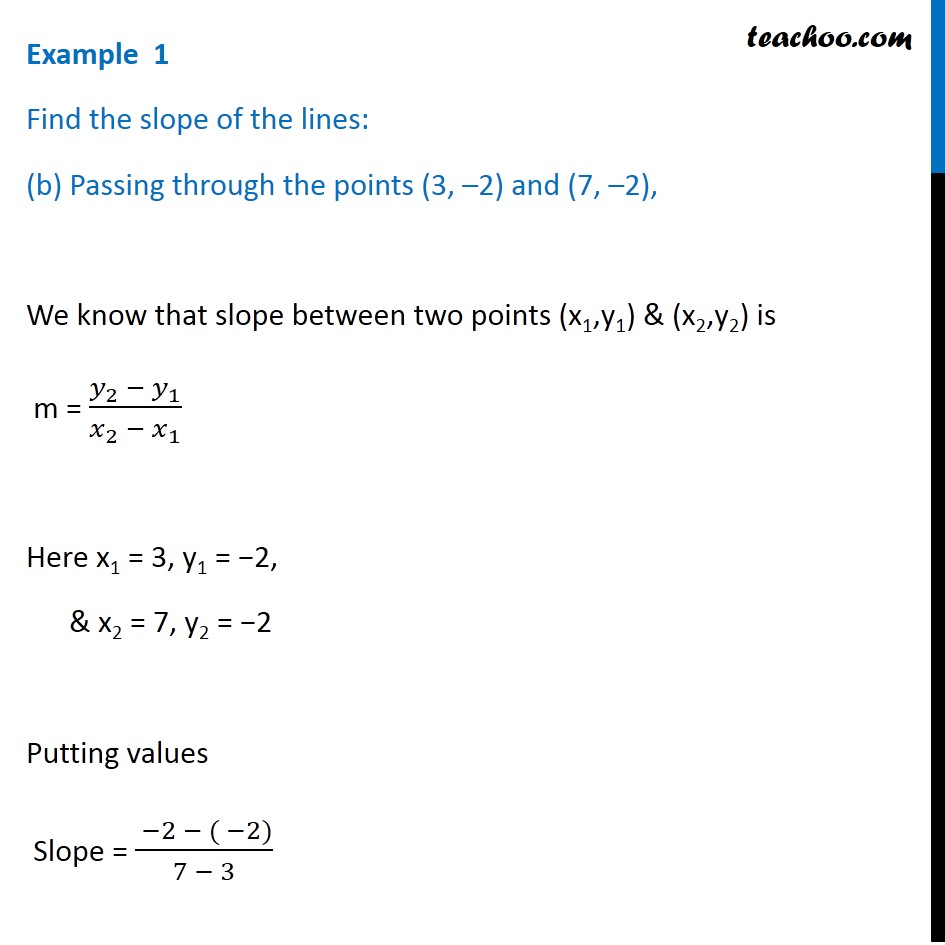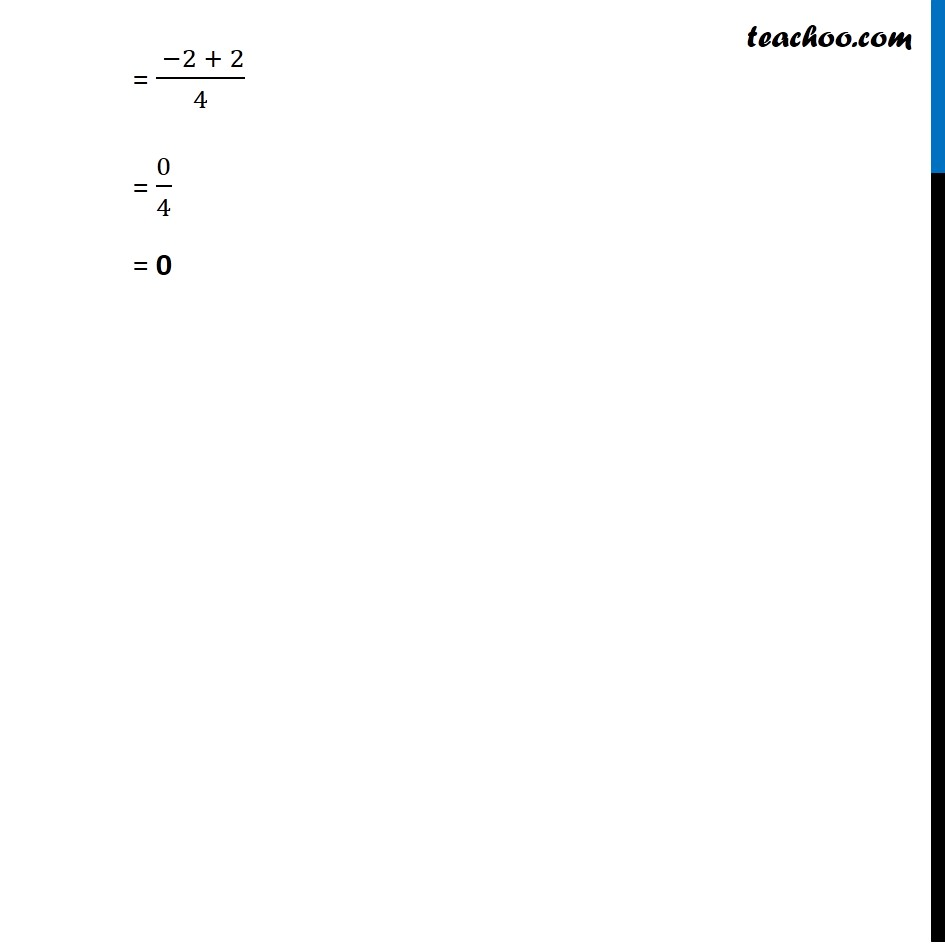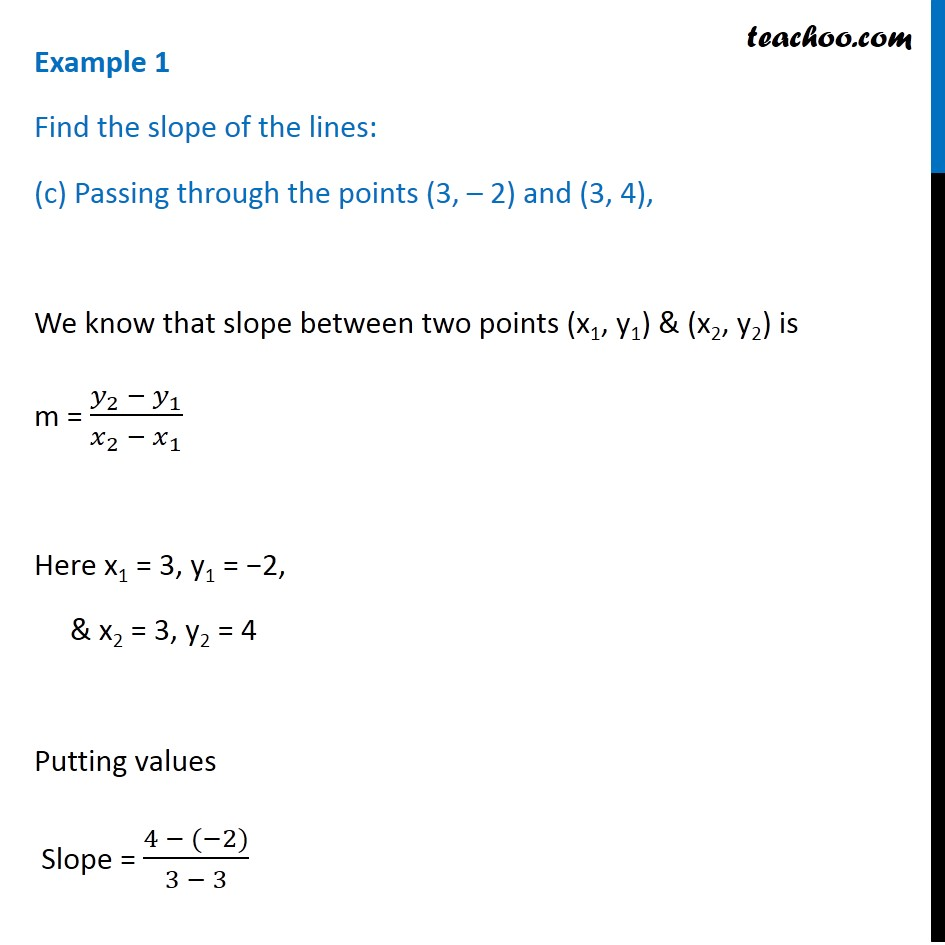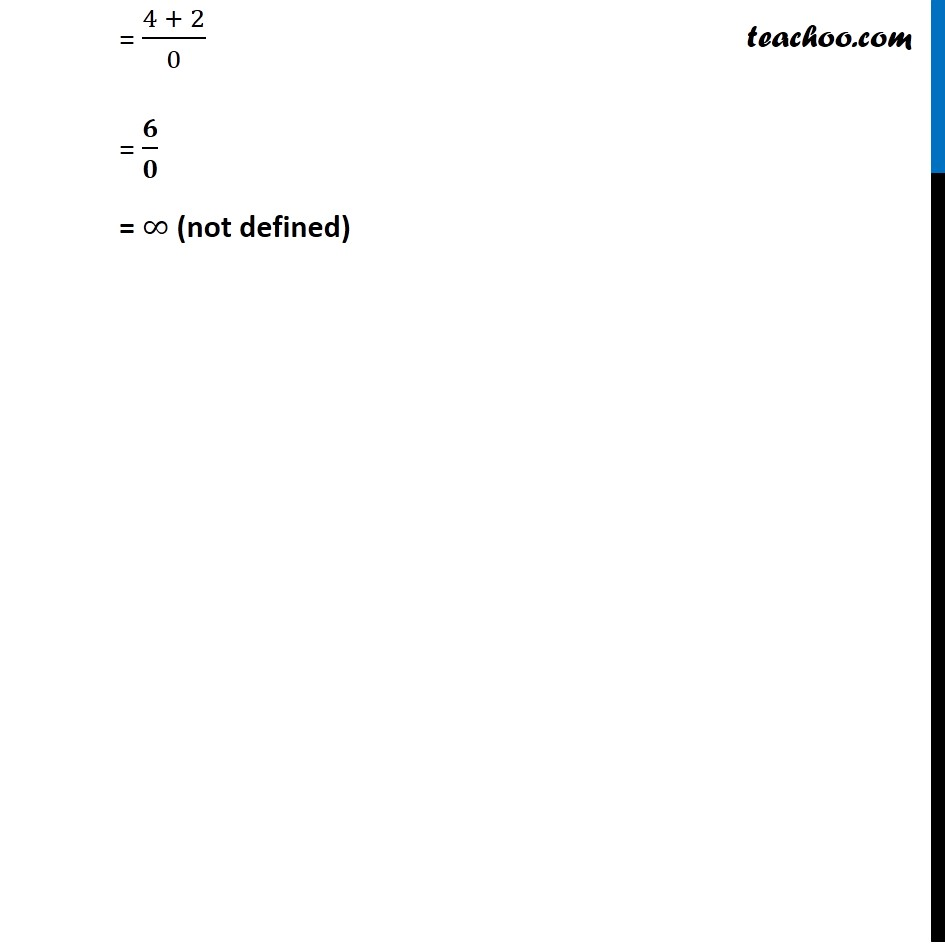Subscribe to our Youtube Channel - https://you.tube/teachoo

1. Chapter 10 Class 11 Straight Lines
2. Serial order wise
3. Examples

Transcript

Example 1 Find the slope of the lines: Passing through the points (3, –2) and (–1, 4) We know that slope between two points (x1, y1) & (x2, y2) is m = (𝑦_2 − 𝑦_1)/(𝑥_2 − 𝑥_1 ) Here x1 = 3, y1 = −2, & x2 = −1, y2 = 4 Putting values Slope = (4 − (−2))/(−1 − 3) = (4 + 2)/( −4) = 6/( −4) = ( −𝟑)/𝟐 Example 1 Find the slope of the lines: (b) Passing through the points (3, –2) and (7, –2), We know that slope between two points (x1,y1) & (x2,y2) is m = (𝑦_2 − 𝑦_1)/(𝑥_2 − 𝑥_1 ) Here x1 = 3, y1 = −2, & x2 = 7, y2 = −2 Putting values Slope = ( −2 − ( −2))/(7 − 3) = ( −2 + 2)/4 = 0/4 = 0 Example 1 Find the slope of the lines: (c) Passing through the points (3, – 2) and (3, 4), We know that slope between two points (x1, y1) & (x2, y2) is m = (𝑦_2 − 𝑦_1)/(𝑥_2 − 𝑥_1 ) Here x1 = 3, y1 = −2, & x2 = 3, y2 = 4 Putting values Slope = (4 − (−2))/(3 − 3) = (4 + 2)/0 = 𝟔/𝟎 = ∞ (not defined) Example 1 Find the slope of the lines: (d) Making inclination of 60° with the positive direction of x-axis. Slope = m = tan θ Given 𝜃 = 60° Hence, m = tan θ = tan 60° = √𝟑

Examples